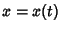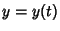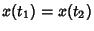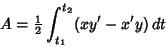## Isoperimetric Problem

Find a closed plane curve of a given length which encloses the greatest Area. The solution is a Circle. If the class of curves to be considered is limited to smooth curves, the isoperimetric problem can be stated symbolically as follows: find an arc with parametric equations,forsuch that,(where no further intersections occur) constrained bysuch thatis a Maximum.

Bogomolny, A. Isoperimetric Theorem and Inequality.'' http://www.cut-the-knot.com/do_you_know/isoperimetric.html.